# 2.3 Generalized dynamic programming for multiple sequence alignment ¶

Until now we worked with alignments between two sequences, but it is likely that you will want to align many sequences at the same time. For example, if you are trying to gain insight on the evolutionary relationships between all of the 16S bacterial genes in a given sample, it would be time consuming and very inefficient to compare them two at a time. It would be more efficient and useful to compare all of the 16S sequences from the bacteria in the same alignment. In the pairwise sequence alignment chapter, we went over dynamic programming algorithms. It's possible to generalize Smith-Waterman and Needleman-Wunsch, the dynamic programming algorithms that we explored for pairwise sequence alignment, to identify the optimal alignment of more than two sequences. Remember that our scoring scheme for pairwise alignment with Smith-Waterman looked like the following:

\begin{align} & F(0, 0) = 0\\ & F(i, 0) = F(i-1, 0) - d\\ & F(0, j) = F(0, j-1) - d\\ \\ & F(i, j) = max \begin{pmatrix} & F(i-1, j-1) + s(c_i, c_j)\\ & F(i-1, j) - d\\ & F(i, j-1) - d)\\ \end{pmatrix} \end{align}

To generalize this to three sequences, we could create $3 \times 3$ scoring, dynamic programming, and traceback matrices. Our scoring scheme would then look like the following:

\begin{align} & F(0, 0, 0) = 0\\ & F(i, 0, 0) = F(i-1, 0, 0) - d\\ & F(0, j, 0) = F(0, j-1, 0) - d\\ & F(0, 0, k) = F(0, 0, k-1) - d\\ \\ & F(i, j, k) = max \begin{pmatrix} F(i-1, j-1, k-1) + s(c_i, c_j) + s(c_i, c_k) + s(c_j, c_k)\\ F(i, j-1, k-1) + s(c_j, c_k) - d\\ F(i-1, j, k-1) + s(c_i, c_k) - d\\ F(i-1, j-1, k) + s(c_i, c_j) - d\\ F(i, j, k-1) - 2d\\ F(i, j-1, k) - 2d\\ F(i-1, j, k) - 2d\\ \end{pmatrix} \end{align}

However the complexity of this algorithm is much worse than for pairwise alignment. For pairwise alignment, remember that if aligning two sequences of lengths $m$ and $n$, the runtime of the algorithm will be proportional to $m \times n$. If $n$ is longer than or as long as $m$, we simplify the statement to say that the runtime of the algorithm will be be proportional to $n^2$. This curve has a pretty scary trajectory: runtime for pairwise alignment with dynamic programming is said to scale quadratically.

In :
%pylab inline
from functools import partial
from IPython.core import page
page.page = print

Populating the interactive namespace from numpy and matplotlib

In :
import matplotlib.pyplot as plt

seq_lengths = range(25)
s2_times = [t ** 2 for t in range(25)]

plt.plot(range(25), s2_times)
plt.xlabel('Sequence Length')
plt.ylabel('Runtime (s)')

Out:
Text(0,0.5,'Runtime (s)')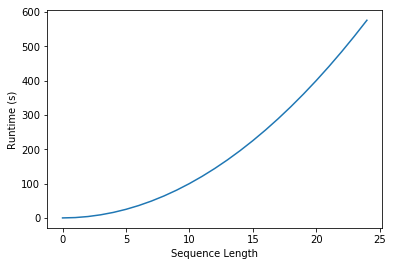The exponent in the $n^2$ term comes from the fact that, in pairwise alignment, if we assume our sequences are both of length $n$, there are $n \times n$ cells to fill in in the dynamic programming matrix. If we were to generalize either Smith-Waterman or Needleman-Wunsch to three sequences, we would need to create a 3 dimensional array to score and trace back the alignment. For sequences of length $n$, we would therefore have $n \times n \times n$ cells to fill in, and our runtime versus sequence length curve would look like the following.

In :
s3_times = [t ** 3 for t in range(25)]

plt.plot(range(25), s3_times)
plt.xlabel('Sequence Length')
plt.ylabel('Runtime (s)')

Out:
Text(0,0.5,'Runtime (s)')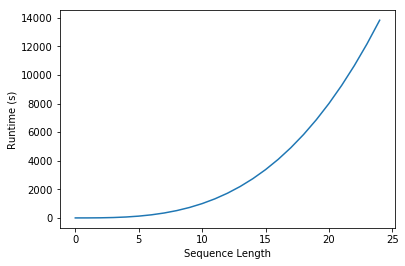That curve looks steeper than the curve for pairwise alignment, and the values on the y-axis are bigger, but it's not really clear how much of a problem this is until we plot runtime for three sequences in the context of the run times for pairwise alignment.

In :
plt.plot(range(25), s2_times)
plt.plot(range(25), s3_times)
plt.xlabel('Sequence Length')
plt.ylabel('Runtime (s)')

Out:
Text(0,0.5,'Runtime (s)')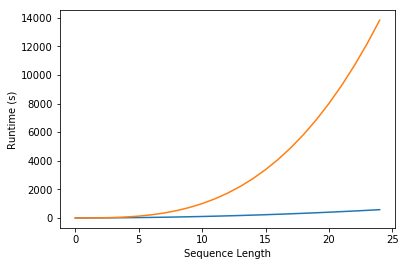And for four sequences:

In :
s4_times = [t ** 4 for t in range(25)]

plt.plot(range(25), s2_times)
plt.plot(range(25), s3_times)
plt.plot(range(25), s4_times)
plt.xlabel('Sequence Length')
plt.ylabel('Runtime (s)')

Out:
Text(0,0.5,'Runtime (s)')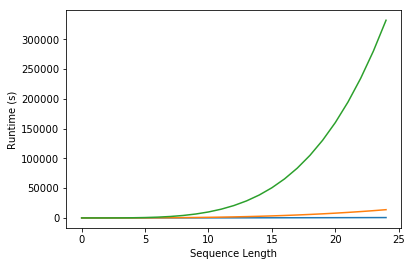We clearly have a problem here, and that is that the runtime for multiple sequence alignment using full dynamic programming algorithms grows exponentially with the number of sequences to be aligned. If $n$ is our sequence length, and $s$ is the number of sequences, that means that runtime is proportional to $n^s$. In pairwise alignment, $s$ is always equal to 2, so the problem is more manageable. However, for the general case of $s$ sequences, we really can't even consider Smith-Waterman or Needleman-Wunsch for more than just a few sequences. The pattern in the plots above should illustrate why.

As we explored with database searching, we need to figure out how to align fewer sequences. This is where progressive alignment comes in.

## 2.3.1 Progressive alignment¶

In progressive alignment, the problem of exponential growth of runtime and space is managed by selectively aligning pairs of sequences, and aligning alignments of sequences. What we typically do is identify a pair of closely related sequences, and align those. Then, we identify the next most closely related sequence to that initial pair, and align that sequence to the alignment. This concept of aligning a sequence to an alignment is new, and we'll come back to it in just a few minutes. The other concept of identifying the most closely related sequences, and then the next most closely related sequence, and so on should sound familiar. It effectively means that we're traversing a tree. And herein lies our problem: we need a tree to efficiently align multiple sequences, but we need an alignment to build a good tree.

You probably have two burning questions in your mind right now:

1. How do we build a tree to guide the alignment process, if we need an alignment to build a good tree?
2. How do we align a sequence to an alignment, or an alignment to an alignment?

We'll explore both of those through-out the rest of this notebook. First, let's cover the process of progressive multiple sequence alignment, just assuming for a moment that we know how to do both of those things.

The process of progressive multiple sequence alignment could look like the following. First, we start with some sequences and a tree representing the relationship between those sequences. We'll call this our guide tree, because it's going to guide us through the process of multiple sequence alignment. In progressive multiple sequence alignment, we build a multiple sequence alignment for each internal node of the tree, where the alignment at a given internal node contains all of the sequences in the clade defined by that node.Starting from the root node, descend the bottom branch of the tree until you get to the an internal node. If an alignment hasn't been constructed for that node yet, continue descending the tree until to get to a pair of nodes. In this case, we follow the two branches to the tips. We then align the sequences at that pair of tips (usually with Needleman-Wunsch, for multiple sequence alignment), and assign that alignment to the node connecting those tips.Next, we want to find what to align the resulting alignment to, so start from the root node and descend the top branch of the tree. When you get to the next node, determine if an alignment has already been created for that node. If not, our job is to build that alignment so we have something to align against. In this case, that means that we need to align s1, s2, and s3. We can achieve this by aligning s1 and s3 first, to get the alignment at the internal node connecting them.We can next align the alignment of s1 and s3 with s2, to get the alignment at the internal node connecting those clades.And finally, we can compute the alignment at the root node of the tree, by aligning the alignment of s1, s2, and s3 with the alignment of s4 and s5.The alignment at the root node is our multiple sequence alignment.

### 2.3.1.1 Building the guide tree¶

Let's address the first of our outstanding questions. I mentioned above that we need an alignment to build a good tree. The key word here is good. We can build a very rough tree - one that we would never want to present as representing the actual relationships between the sequences in question - without first aligning the sequences. Remember that building a UPGMA tree requires only a distance matrix, so if we can find a non-alignment-dependent way to compute distances between the sequences, we can build a rough UPGMA tree from them.

Let's compute distances between the sequences based on their word composition. We'll define a word here as k adjacent characters in the sequence. We can then define a function that will return all of the words in a sequence as follows. These words can be defined as being overlapping, or non-overlapping. We'll go with overlapping for this example, as the more words we have, the better our guide tree should be.

In :
from skbio import DNA
%psource DNA.iter_kmers

    @stable(as_of="0.4.0")
def iter_kmers(self, k, overlap=True):
"""Generate kmers of length k from this sequence.

Parameters
----------
k : int
The kmer length.
overlap : bool, optional
Defines whether the kmers should be overlapping or not.

Yields
------
Sequence
kmer of length k contained in this sequence.

Raises
------
ValueError
If k is less than 1.

Examples
--------
>>> from skbio import Sequence
>>> s = Sequence('ACACGACGTT')
>>> for kmer in s.iter_kmers(4, overlap=False):
...     str(kmer)
'ACAC'
'GACG'
>>> for kmer in s.iter_kmers(3, overlap=True):
...     str(kmer)
'ACA'
'CAC'
'ACG'
'CGA'
'GAC'
'ACG'
'CGT'
'GTT'

"""
if k < 1:
raise ValueError("k must be greater than 0.")

if overlap:
step = 1
count = len(self) - k + 1
else:
step = k
count = len(self) // k

if self.has_positional_metadata():
for i in range(0, len(self) - k + 1, step):
yield self[i:i+k]
# Optimized path when no positional metadata
else:
kmers = np.lib.stride_tricks.as_strided(
self._bytes, shape=(k, count), strides=(1, step)).T
for s in kmers:
yield self._to(sequence=s)


In :
for e in DNA("ACCGGTGACCAGTTGACCAGTA").iter_kmers(3):
print(e)

ACC
CCG
CGG
GGT
GTG
TGA
GAC
ACC
CCA
CAG
AGT
GTT
TTG
TGA
GAC
ACC
CCA
CAG
AGT
GTA

In :
for e in DNA("ACCGGTGACCAGTTGACCAGTA").iter_kmers(7):
print(e)

ACCGGTG
CCGGTGA
CGGTGAC
GGTGACC
GTGACCA
TGACCAG
GACCAGT
ACCAGTT
CCAGTTG
CAGTTGA
AGTTGAC
GTTGACC
TTGACCA
TGACCAG
GACCAGT
ACCAGTA

In :
for e in DNA("ACCGGTGACCAGTTGACCAGTA").iter_kmers(3, overlap=False):
print(e)

ACC
GGT
GAC
CAG
TTG
ACC
AGT


If we then have two sequences, we can compute the word counts for each and define a distance between the sequences as the fraction of words that are unique to either sequence.

In :
from iab.algorithms import kmer_distance
%psource kmer_distance

def kmer_distance(sequence1, sequence2, k=3, overlap=True):
"""Compute the kmer distance between a pair of sequences

Parameters
----------
sequence1 : skbio.Sequence
sequence2 : skbio.Sequence
k : int, optional
The word length.
overlapping : bool, optional
Defines whether the k-words should be overlapping or not
overlapping.

Returns
-------
float
Fraction of the set of k-mers from both sequence1 and
sequence2 that are unique to either sequence1 or
sequence2.

Raises
------
ValueError
If k < 1.

Notes
-----
k-mer counts are not incorporated in this distance metric.

"""
sequence1_kmers = set(map(str, sequence1.iter_kmers(k, overlap)))
sequence2_kmers = set(map(str, sequence2.iter_kmers(k, overlap)))
all_kmers = sequence1_kmers | sequence2_kmers
shared_kmers = sequence1_kmers & sequence2_kmers
number_unique = len(all_kmers) - len(shared_kmers)
fraction_unique = number_unique / len(all_kmers)
return fraction_unique



We can then use this as a distance function...

In :
s1 = DNA("ACCGGTGACCAGTTGACCAGT")
s2 = DNA("ATCGGTACCGGTAGAAGT")
s3 = DNA("GGTACCAAATAGAA")

print(s1.distance(s2, kmer_distance))
print(s1.distance(s3, kmer_distance))

0.75
0.8571428571428571


If we wanted to override the default to create (for example) a 5-mer distance function, we could use functools.partial.

In :
fivemer_distance = partial(kmer_distance, k=5)

s1 = DNA("ACCGGTGACCAGTTGACCAGT")
s2 = DNA("ATCGGTACCGGTAGAAGT")
s3 = DNA("GGTACCAAATAGAA")

print(s1.distance(s2, fivemer_distance))
print(s1.distance(s3, fivemer_distance))

0.9166666666666666
1.0


We can now apply one of these functions to build a distance matrix for a set of sequences that we want to align.

In :
query_sequences = [DNA("ACCGGTGACCAGTTGACCAGT", {"id": "s1"}),
DNA("ATCGGTACCGGTAGAAGT", {"id": "s2"}),
DNA("GGTACCAAATAGAA", {"id": "s3"}),
DNA("GGCACCAAACAGAA", {"id": "s4"}),
DNA("GGCCCACTGAT", {"id": "s5"})]

In :
from skbio import DistanceMatrix

guide_dm = DistanceMatrix.from_iterable(query_sequences, metric=kmer_distance, key='id')


scikit-bio also has some basic visualization functionality for these objects. For example, we can easily visualize this object as a heatmap.

In :
fig = guide_dm.plot(cmap='Greens')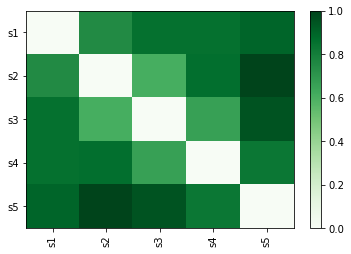We can next use some functionality from SciPy to cluster the sequences with UPGMA, and print out a dendrogram.

In :
from scipy.cluster.hierarchy import average, dendrogram, to_tree

for q in query_sequences:
print(q)

guide_lm = average(guide_dm.condensed_form())
guide_d = dendrogram(guide_lm, labels=guide_dm.ids, orientation='right',
link_color_func=lambda x: 'black')
guide_tree = to_tree(guide_lm)

ACCGGTGACCAGTTGACCAGT
ATCGGTACCGGTAGAAGT
GGTACCAAATAGAA
GGCACCAAACAGAA
GGCCCACTGAT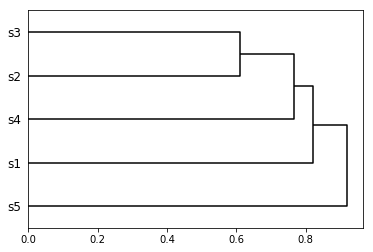In :
from iab.algorithms import guide_tree_from_sequences
%psource guide_tree_from_sequences

def guide_tree_from_sequences(sequences,
metric=kmer_distance,
display_tree = False):
""" Build a UPGMA tree by applying metric to sequences

Parameters
----------
sequences : list of skbio.Sequence objects (or subclasses)
The sequences to be represented in the resulting guide tree.
metric : function
Function that returns a single distance value when given a pair of
skbio.Sequence objects.
display_tree : bool, optional
Print the tree before returning.

Returns
-------
skbio.TreeNode

"""
guide_dm = DistanceMatrix.from_iterable(
sequences, metric=metric, key='id')
guide_lm = sp.cluster.hierarchy.average(guide_dm.condensed_form())
guide_tree = to_tree(guide_lm)
if display_tree:
guide_d = sp.cluster.hierarchy.dendrogram(guide_lm, labels=guide_dm.ids, orientation='right',
link_color_func=lambda x: 'black')
return guide_tree


In :
t = guide_tree_from_sequences(query_sequences, display_tree=True)We now have a guide tree, so we can move on to the next step of progressive alignment.

### 2.3.1.2 Generalization of Needleman-Wunsch (with affine gap scoring) for progressive multiple sequence alignment¶

Next, we'll address our second burning question: aligning alignments. As illustrated above, there are basically three different types of pairwise alignment we need to support for progressive multiple sequence alignment with Needleman-Wunsch. These are:

1. Alignment of a pair of sequences.
2. Alignment of a sequence and an alignment.
3. Alignment of a pair of alignments.

Standard Needleman-Wunsch supports the first, and it is very easy to generalize it to support the latter two. The only change that is necessary is in how the alignment of two non-gap characters is scored. Recall that we previously scored an alignment of two characters by looking up the score of substitution from one to the other in a substitution matrix. To adapt this for aligning a sequence to an alignment, or for aligning an alignment to an alignment, we compute this substitution as the average score of aligning the pairs of characters.

For example, if we want to align the alignment column from $aln1$:

A
C

to the alignment column from $aln2$:

T
G

we could compute the substitution score using the matrix $m$ as:

$$s = \frac{m[A][T] + m[A][G] + m[C][T] + m[C][G]}{aln1_{length} \times aln2_{length}}$$

The following code adapts our implementation of Needleman-Wunsch to support aligning a sequence to an alignment, or aligning an alignment to an alignment.

In :
from iab.algorithms import format_dynamic_programming_matrix, format_traceback_matrix
from skbio.alignment._pairwise import _compute_score_and_traceback_matrices

%psource _compute_score_and_traceback_matrices

def _compute_score_and_traceback_matrices(
aln1, aln2, gap_open_penalty, gap_extend_penalty, substitution_matrix,
new_alignment_score=-np.inf, init_matrices_f=_init_matrices_nw,
penalize_terminal_gaps=True, gap_substitution_score=0):
"""Return dynamic programming (score) and traceback matrices.

A note on the penalize_terminal_gaps parameter. When this value is
False, this function is no longer true Smith-Waterman/Needleman-Wunsch
scoring, but when True it can result in biologically irrelevant
artifacts in Needleman-Wunsch (global) alignments. Specifically, if one
sequence is longer than the other (e.g., if aligning a primer sequence to
an amplification product, or searching for a gene in a genome) the shorter
sequence will have a long gap inserted. The parameter is True by
default (so that this function computes the score and traceback matrices as
described by the original authors) but the global alignment wrappers pass
False by default, so that the global alignment API returns the result
that users are most likely to be looking for.

"""
aln1_length = aln1.shape.position
aln2_length = aln2.shape.position
# cache some values for quicker/simpler access
aend = _traceback_encoding['alignment-end']
match = _traceback_encoding['match']
vgap = _traceback_encoding['vertical-gap']
hgap = _traceback_encoding['horizontal-gap']

new_alignment_score = (new_alignment_score, aend)

# Initialize a matrix to use for scoring the alignment and for tracing
# back the best alignment
score_matrix, traceback_matrix = init_matrices_f(
aln1, aln2, gap_open_penalty, gap_extend_penalty)

# Iterate over the characters in aln2 (which corresponds to the vertical
# sequence in the matrix)
for aln2_pos, aln2_chars in enumerate(aln2.iter_positions(), 1):
aln2_chars = str(aln2_chars)

# Iterate over the characters in aln1 (which corresponds to the
# horizontal sequence in the matrix)
for aln1_pos, aln1_chars in enumerate(aln1.iter_positions(), 1):
aln1_chars = str(aln1_chars)

# compute the score for a match/mismatch
substitution_score = _compute_substitution_score(
aln1_chars, aln2_chars, substitution_matrix,
gap_substitution_score, aln1.dtype.gap_chars)

diag_score = \
(score_matrix[aln2_pos-1, aln1_pos-1] + substitution_score,
match)

# compute the score for adding a gap in aln2 (vertical)
if not penalize_terminal_gaps and (aln1_pos == aln1_length):
# we've reached the end of aln1, so adding vertical gaps
# (which become gaps in aln1) should no longer
# be penalized (if penalize_terminal_gaps == False)
up_score = (score_matrix[aln2_pos-1, aln1_pos], vgap)
elif traceback_matrix[aln2_pos-1, aln1_pos] == vgap:
# gap extend, because the cell above was also a gap
up_score = \
(score_matrix[aln2_pos-1, aln1_pos] - gap_extend_penalty,
vgap)
else:
# gap open, because the cell above was not a gap
up_score = \
(score_matrix[aln2_pos-1, aln1_pos] - gap_open_penalty,
vgap)

# compute the score for adding a gap in aln1 (horizontal)
if not penalize_terminal_gaps and (aln2_pos == aln2_length):
# we've reached the end of aln2, so adding horizontal gaps
# (which become gaps in aln2) should no longer
# be penalized (if penalize_terminal_gaps == False)
left_score = (score_matrix[aln2_pos, aln1_pos-1], hgap)
elif traceback_matrix[aln2_pos, aln1_pos-1] == hgap:
# gap extend, because the cell to the left was also a gap
left_score = \
(score_matrix[aln2_pos, aln1_pos-1] - gap_extend_penalty,
hgap)
else:
# gap open, because the cell to the left was not a gap
left_score = \
(score_matrix[aln2_pos, aln1_pos-1] - gap_open_penalty,
hgap)

# identify the largest score, and use that information to populate
# the score and traceback matrices
best_score = _first_largest([new_alignment_score, left_score,
diag_score, up_score])
score_matrix[aln2_pos, aln1_pos] = best_score
traceback_matrix[aln2_pos, aln1_pos] = best_score

return score_matrix, traceback_matrix


In :
from skbio.alignment._pairwise import _traceback
%psource _traceback

def _traceback(traceback_matrix, score_matrix, aln1, aln2, start_row,
start_col):
# cache some values for simpler reference
aend = _traceback_encoding['alignment-end']
match = _traceback_encoding['match']
vgap = _traceback_encoding['vertical-gap']
hgap = _traceback_encoding['horizontal-gap']
gap_character = aln1.dtype.default_gap_char

# initialize the result alignments
aln1_sequence_count = aln1.shape.sequence
aligned_seqs1 = [[] for e in range(aln1_sequence_count)]

aln2_sequence_count = aln2.shape.sequence
aligned_seqs2 = [[] for e in range(aln2_sequence_count)]

current_row = start_row
current_col = start_col

best_score = score_matrix[current_row, current_col]
current_value = None

while current_value != aend:
current_value = traceback_matrix[current_row, current_col]

if current_value == match:
for aligned_seq, input_seq in zip(aligned_seqs1, aln1):
aligned_seq.append(str(input_seq[current_col-1]))
for aligned_seq, input_seq in zip(aligned_seqs2, aln2):
aligned_seq.append(str(input_seq[current_row-1]))
current_row -= 1
current_col -= 1
elif current_value == vgap:
for aligned_seq in aligned_seqs1:
aligned_seq.append(gap_character)
for aligned_seq, input_seq in zip(aligned_seqs2, aln2):
aligned_seq.append(str(input_seq[current_row-1]))
current_row -= 1
elif current_value == hgap:
for aligned_seq, input_seq in zip(aligned_seqs1, aln1):
aligned_seq.append(str(input_seq[current_col-1]))
for aligned_seq in aligned_seqs2:
aligned_seq.append(gap_character)
current_col -= 1
elif current_value == aend:
continue
else:
raise ValueError(
"Invalid value in traceback matrix: %s" % current_value)

for i in range(aln1_sequence_count):
aligned_seq = ''.join(aligned_seqs1[i][::-1])
constructor = aln1.dtype
aligned_seqs1[i] = constructor(aligned_seq)

for i in range(aln2_sequence_count):
aligned_seq = ''.join(aligned_seqs2[i][::-1])
constructor = aln2.dtype
aligned_seqs2[i] = constructor(aligned_seq)

return aligned_seqs1, aligned_seqs2, best_score, current_col, current_row


In :
from skbio.alignment import global_pairwise_align_nucleotide
%psource global_pairwise_align_nucleotide

@experimental(as_of="0.4.0")
def global_pairwise_align_nucleotide(seq1, seq2, gap_open_penalty=5,
gap_extend_penalty=2,
match_score=1, mismatch_score=-2,
substitution_matrix=None,
penalize_terminal_gaps=False):
"""Globally align nucleotide seqs or alignments with Needleman-Wunsch

Parameters
----------
seq1 : DNA, RNA, or TabularMSA[DNA|RNA]
The first unaligned sequence(s).
seq2 : DNA, RNA, or TabularMSA[DNA|RNA]
The second unaligned sequence(s).
gap_open_penalty : int or float, optional
Penalty for opening a gap (this is substracted from previous best
alignment score, so is typically positive).
gap_extend_penalty : int or float, optional
Penalty for extending a gap (this is substracted from previous best
alignment score, so is typically positive).
match_score : int or float, optional
The score to add for a match between a pair of bases (this is added
to the previous best alignment score, so is typically positive).
mismatch_score : int or float, optional
The score to add for a mismatch between a pair of bases (this is
added to the previous best alignment score, so is typically
negative).
substitution_matrix: 2D dict (or similar)
Lookup for substitution scores (these values are added to the
previous best alignment score). If provided, this overrides
match_score and mismatch_score.
penalize_terminal_gaps: bool, optional
If True, will continue to penalize gaps even after one sequence has
been aligned through its end. This behavior is true Needleman-Wunsch
alignment, but results in (biologically irrelevant) artifacts when
the sequences being aligned are of different length. This is False
by default, which is very likely to be the behavior you want in all or
nearly all cases.

Returns
-------
tuple
TabularMSA object containing the aligned sequences, alignment score
(float), and start/end positions of each input sequence (iterable
of two-item tuples). Note that start/end positions are indexes into the
unaligned sequences.

See Also
--------
local_pairwise_align
local_pairwise_align_protein
local_pairwise_align_nucleotide
skbio.alignment.local_pairwise_align_ssw
global_pairwise_align
global_pairwise_align_protein

Notes
-----
Default match_score, mismatch_score, gap_open_penalty and
gap_extend_penalty parameters are derived from the NCBI BLAST
Server _.

This function can be use to align either a pair of sequences, a pair of
alignments, or a sequence and an alignment.

References
----------
..  http://blast.ncbi.nlm.nih.gov/Blast.cgi

"""
for seq in seq1, seq2:
if not isinstance(seq, (DNA, RNA, TabularMSA)):
raise TypeError(
"seq1 and seq2 must be DNA, RNA, or TabularMSA, not type "
"%r" % type(seq).__name__)
if isinstance(seq, TabularMSA) and not issubclass(seq.dtype,
(DNA, RNA)):
raise TypeError(
"seq1 and seq2 must be TabularMSA with DNA or RNA dtype, "
"not dtype %r" % seq.dtype.__name__)

# use the substitution matrix provided by the user, or compute from
# match_score and mismatch_score if a substitution matrix was not provided
if substitution_matrix is None:
substitution_matrix = \
make_identity_substitution_matrix(match_score, mismatch_score)

return global_pairwise_align(seq1, seq2, gap_open_penalty,
gap_extend_penalty, substitution_matrix,
penalize_terminal_gaps=penalize_terminal_gaps)



For the sake of the examples below, I'm going to override one of the global_pairwise_align_nucleotide defaults to penalize terminal gaps. This effectively tells the algorithm that we know we have a collection of sequences that are homologous from beginning to end.

In :
global_pairwise_align_nucleotide = partial(global_pairwise_align_nucleotide, penalize_terminal_gaps=True)


For example, we can still use this code to align pairs of sequences (but note that we now need to pass those sequences in as a pair of one-item lists):

In :
aln1, _, _ = global_pairwise_align_nucleotide(query_sequences, query_sequences)
print(aln1)

TabularMSA[DNA]
----------------------
Stats:
sequence count: 2
position count: 21
----------------------
ACCGGTGACCAGTTGACCAGT
ATCGGT-ACCGGTAGA--AGT

/tmp/build/1712b08a/iab-env/lib/python3.5/site-packages/skbio/alignment/_pairwise.py:601: EfficiencyWarning: You're using skbio's python implementation of Needleman-Wunsch alignment. This is known to be very slow (e.g., thousands of times slower than a native C implementation). We'll be adding a faster version soon (see https://github.com/biocore/scikit-bio/issues/254 to track progress on this).
"to track progress on this).", EfficiencyWarning)


We can align that alignment to one of our other sequences.

In :
aln1, _, _ = global_pairwise_align_nucleotide(aln1, query_sequences)
print(aln1)

TabularMSA[DNA]
----------------------
Stats:
sequence count: 3
position count: 21
----------------------
ACCGGTGACCAGTTGACCAGT
ATCGGT-ACCGGTAGA--AGT
---GGTACCAAATAGA--A--


Alternatively, we can align another pair of sequences:

In :
aln2, _, _ = global_pairwise_align_nucleotide(query_sequences, query_sequences)
print(aln2)

TabularMSA[DNA]
----------------------
Stats:
sequence count: 2
position count: 14
----------------------
GGTACCAAATAGAA
GGCACCAAACAGAA


And then align that alignment against our previous alignment:

In :
aln3, _, _  = global_pairwise_align_nucleotide(aln1, aln2)
print(aln3)

TabularMSA[DNA]
----------------------
Stats:
sequence count: 5
position count: 21
----------------------
ACCGGTGACCAGTTGACCAGT
ATCGGT-ACCGGTAGA--AGT
---GGTACCAAATAGA--A--
---GGTACCAAATAGA----A
---GGCACCAAACAGA----A


### 2.3.1.3 Putting it all together: progressive multiple sequence alignment¶

We can now combine all of these steps to take a set of query sequences, build a guide tree, perform progressive multiple sequence alignment, and return the guide tree (as a SciPy linkage matrix) and the alignment.

In :
from skbio import TreeNode
guide_tree = TreeNode.from_linkage_matrix(guide_lm, guide_dm.ids)


We can view the guide tree in Newick format as follows:

In :
print(guide_tree)

(s5:0.45975877193,(s1:0.410714285714,(s4:0.382575757576,(s2:0.305555555556,s3:0.305555555556):0.0770202020202):0.0281385281385):0.0490444862155);


In :
from iab.algorithms import progressive_msa
%psource progressive_msa

def progressive_msa(sequences, pairwise_aligner, guide_tree=None,
metric=kmer_distance):
""" Perform progressive msa of sequences

Parameters
----------
sequences : skbio.SequenceCollection
The sequences to be aligned.
metric : function, optional
Function that returns a single distance value when given a pair of
skbio.Sequence objects. This will be used to build a guide tree if one
is not provided.
guide_tree : skbio.TreeNode, optional
The tree that should be used to guide the alignment process.
pairwise_aligner : function
Function that should be used to perform the pairwise alignments,
for example skbio.alignment.global_pairwise_align_nucleotide. Must
support skbio.Sequence objects or skbio.TabularMSA objects
as input.

Returns
-------
skbio.TabularMSA

"""

if guide_tree is None:
guide_dm = DistanceMatrix.from_iterable(
sequences, metric=metric, key='id')
guide_lm = sp.cluster.hierarchy.average(guide_dm.condensed_form())
guide_tree = TreeNode.from_linkage_matrix(guide_lm, guide_dm.ids)

seq_lookup = {s.metadata['id']: s for i, s in enumerate(sequences)}
c1, c2 = guide_tree.children
if c1.is_tip():
c1_aln = seq_lookup[c1.name]
else:
c1_aln = progressive_msa(sequences, pairwise_aligner, c1)

if c2.is_tip():
c2_aln = seq_lookup[c2.name]
else:
c2_aln = progressive_msa(sequences, pairwise_aligner, c2)

alignment, _, _ = pairwise_aligner(c1_aln, c2_aln)
# this is a temporary hack as the aligners in skbio 0.4.1 are dropping
# metadata - this makes sure that the right metadata is associated with
# the sequence after alignment
if isinstance(c1_aln, Sequence):
alignment.metadata = c1_aln.metadata
len_c1_aln = 1
else:
for i in range(len(c1_aln)):
alignment[i].metadata = c1_aln[i].metadata
len_c1_aln = len(c1_aln)
if isinstance(c2_aln, Sequence):
alignment.metadata = c2_aln.metadata
else:
for i in range(len(c2_aln)):
alignment[len_c1_aln + i].metadata = c2_aln[i].metadata

return alignment


In :
msa = progressive_msa(query_sequences, pairwise_aligner=global_pairwise_align_nucleotide, guide_tree=guide_tree)
print(msa)

TabularMSA[DNA]
----------------------
Stats:
sequence count: 5
position count: 21
----------------------
---GGC--CCA-----CTGAT
ACCGGTGACCAGTTGACCAGT
---GGCACCAAACAGA--A--
ATCGGTACC-GGTAGA--AGT
---GGTACCAAATAGA--A--


We can now build a (hopefully) improved tree from our multiple sequence alignment. First we'll look at our original distance matrix again, and then the distance matrix generated from the progressive multiple sequence alignment.

In :
fig = guide_dm.plot(cmap='Greens')In :
msa_dm = DistanceMatrix.from_iterable(msa, metric=kmer_distance)
fig = msa_dm.plot(cmap='Greens')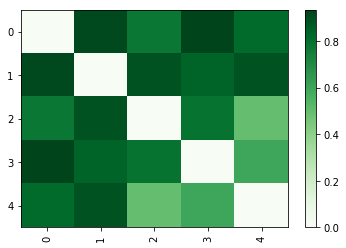The UPGMA trees that result from these alignments are very different. First we'll look at the guide tree, and then the tree resulting from the progressive multiple sequence alignment.

In :
d = dendrogram(guide_lm, labels=guide_dm.ids, orientation='right',
link_color_func=lambda x: 'black')In :
msa_lm = average(msa_dm.condensed_form())
d = dendrogram(msa_lm, labels=msa_dm.ids, orientation='right',
link_color_func=lambda x: 'black')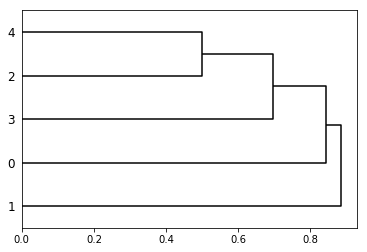And we can wrap this all up in a single convenience function:

In :
from iab.algorithms import progressive_msa_and_tree
%psource progressive_msa_and_tree

def progressive_msa_and_tree(sequences,
pairwise_aligner,
metric=kmer_distance,
guide_tree=None,
display_aln=False,
display_tree=False):
""" Perform progressive msa of sequences and build a UPGMA tree
Parameters
----------
sequences : skbio.SequenceCollection
The sequences to be aligned.
pairwise_aligner : function
Function that should be used to perform the pairwise alignments,
for example skbio.alignment.global_pairwise_align_nucleotide. Must
support skbio.Sequence objects or skbio.TabularMSA objects
as input.
metric : function, optional
Function that returns a single distance value when given a pair of
skbio.Sequence objects. This will be used to build a guide tree if one
is not provided.
guide_tree : skbio.TreeNode, optional
The tree that should be used to guide the alignment process.
display_aln : bool, optional
Print the alignment before returning.
display_tree : bool, optional
Print the tree before returning.

Returns
-------
skbio.alignment
skbio.TreeNode

"""
msa = progressive_msa(sequences, pairwise_aligner=pairwise_aligner,
guide_tree=guide_tree)

if display_aln:
print(msa)

msa_dm = DistanceMatrix.from_iterable(msa, metric=metric, key='id')
msa_lm = sp.cluster.hierarchy.average(msa_dm.condensed_form())
msa_tree = TreeNode.from_linkage_matrix(msa_lm, msa_dm.ids)
if display_tree:
print("\nOutput tree:")
d = sp.cluster.hierarchy.dendrogram(msa_lm, labels=msa_dm.ids, orientation='right',
link_color_func=lambda x: 'black')
return msa, msa_tree


In :
msa = progressive_msa(query_sequences, pairwise_aligner=global_pairwise_align_nucleotide, guide_tree=guide_tree)

In :
msa, tree = progressive_msa_and_tree(query_sequences, pairwise_aligner=global_pairwise_align_nucleotide,
display_tree=True, display_aln=True)

TabularMSA[DNA]
----------------------
Stats:
sequence count: 5
position count: 21
----------------------
---GGC--CCA-----CTGAT
ACCGGTGACCAGTTGACCAGT
---GGCACCAAACAGA--A--
ATCGGTACC-GGTAGA--AGT
---GGTACCAAATAGA--A--

Output tree: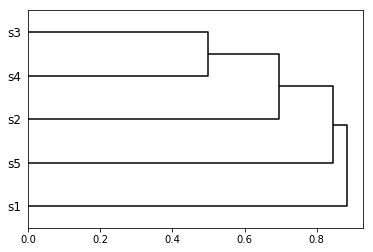## 2.3.2 Progressive alignment versus iterative alignment¶

In an iterative alignment, the output tree from the above progressive alignment is used as a guide tree, and the full process repeated. This is performed to reduce errors that result from a low-quality guide tree.

In :
from iab.algorithms import iterative_msa_and_tree
%psource iterative_msa_and_tree

def iterative_msa_and_tree(sequences,
num_iterations,
pairwise_aligner,
metric=kmer_distance,
display_aln=False,
display_tree=False):
""" Perform progressive msa of sequences and build a UPGMA tree
Parameters
----------
sequences : skbio.SequenceCollection
The sequences to be aligned.
num_iterations : int
The number of iterations of progressive multiple sequence alignment to
perform. Must be greater than zero and less than five.
pairwise_aligner : function
Function that should be used to perform the pairwise alignments,
for example skbio.alignment.global_pairwise_align_nucleotide. Must
support skbio.Sequence objects or skbio.TabularMSA objects
as input.
metric : function, optional
Function that returns a single distance value when given a pair of
skbio.Sequence objects. This will be used to build a guide tree if one
is not provided.
display_aln : bool, optional
Print the alignment before returning.
display_tree : bool, optional
Print the tree before returning.

Returns
-------
skbio.alignment
skbio.TreeNode

"""
if num_iterations > 5:
raise ValueError("A maximum of five iterations is allowed."
"You requested %d." % num_iterations)
previous_iter_tree = None
for i in range(num_iterations):
if i == (num_iterations - 1):
# only display the last iteration
display = True
else:
display = False
previous_iter_msa, previous_iter_tree = \
progressive_msa_and_tree(sequences,
pairwise_aligner=pairwise_aligner,
metric=metric,
guide_tree=previous_iter_tree,
display_aln=display_aln and display,
display_tree=display_tree and display)

return previous_iter_msa, previous_iter_tree


In :
msa, tree = iterative_msa_and_tree(query_sequences, pairwise_aligner=global_pairwise_align_nucleotide, num_iterations=1, display_aln=True, display_tree=True)

TabularMSA[DNA]
----------------------
Stats:
sequence count: 5
position count: 21
----------------------
---GGC--CCA-----CTGAT
ACCGGTGACCAGTTGACCAGT
---GGCACCAAACAGA--A--
ATCGGTACC-GGTAGA--AGT
---GGTACCAAATAGA--A--

Output tree:In :
msa, tree = iterative_msa_and_tree(query_sequences, pairwise_aligner=global_pairwise_align_nucleotide, num_iterations=2, display_aln=True, display_tree=True)

TabularMSA[DNA]
----------------------
Stats:
sequence count: 5
position count: 21
----------------------
ACCGGTGACCAGTTGACCAGT
---GGC--CCACT-----GAT
ATCGGTACC-GGTAGA--AGT
---GGCACCAAACAGA--A--
---GGTACCAAATAGA--A--

Output tree: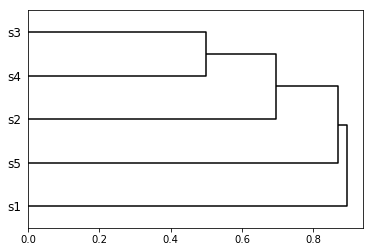In :
msa, tree = iterative_msa_and_tree(query_sequences, pairwise_aligner=global_pairwise_align_nucleotide, num_iterations=3, display_aln=True, display_tree=True)

TabularMSA[DNA]
----------------------
Stats:
sequence count: 5
position count: 21
----------------------
ACCGGTGACCAGTTGACCAGT
---GGC--CCACT-----GAT
ATCGGTACC-GGTAGA--AGT
---GGCACCAAACAGA--A--
---GGTACCAAATAGA--A--

Output tree:In :
msa, tree = iterative_msa_and_tree(query_sequences, pairwise_aligner=global_pairwise_align_nucleotide, num_iterations=5, display_aln=True, display_tree=True)

TabularMSA[DNA]
----------------------
Stats:
sequence count: 5
position count: 21
----------------------
ACCGGTGACCAGTTGACCAGT
---GGC--CCACT-----GAT
ATCGGTACC-GGTAGA--AGT
---GGCACCAAACAGA--A--
---GGTACCAAATAGA--A--

Output tree:Some references that I used in assembling these notes include 1, 2, 3, 4, and 5.